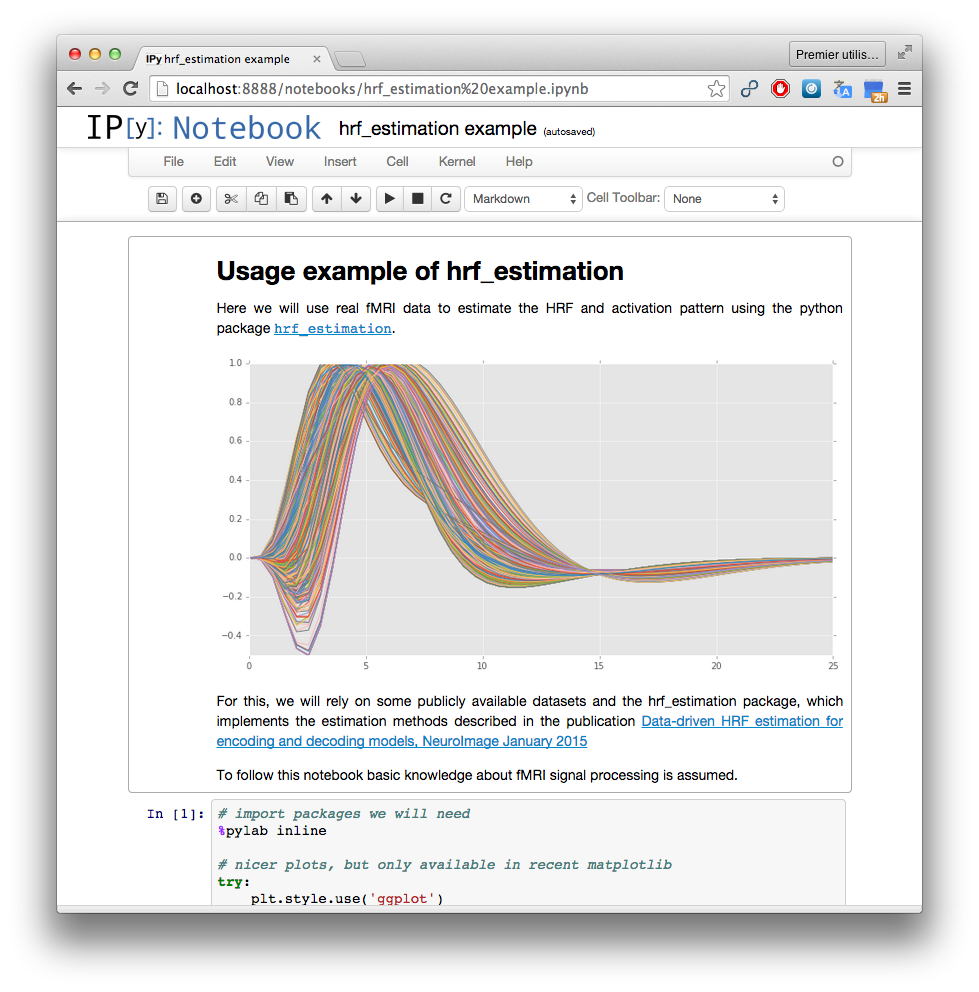#### Next topic

Function signature

# hrf_estimation¶

This package implements method for the joint estimation of hemodynamic response function (HRF) and activation coefficients (aka beta-maps) from fMRI data. The methods implemented here are described in the paper

Data-driven HRF estimation for encoding and decoding models, Pedregosa et al. 2004

# Examples¶The following ipython notebook shows a complete example of how to use the function hrf_estimation.glm() to estimate the HRF and activation coefficients from a giveb BOLD signal.

# Main functions¶

The main function in the package is called hrf_estimation.glm() and will extract the HRF and activation coefficients from BOLD signal.

This function takes as input a vector of conditions, a vector of onsets, the TR (float) and the matrix of BOLD measurements (of size n_timecourse * n_voxels). The return values of hrf_estimation.glm are the estimated HRF, the activation coefficients (a.k.a beta-maps). Note that the estimated HRFs are given as the coefficients in the basis of choice. To obtain the full HRF you must multiply by the basis (the example below shows an example of this)

The function hrf_estimation.glm() is meant to be used whenever the conditions and onsets are available. It will compute internally the design matrix without the possibility to modify it.

However, if you have already formed the design matrix and only want to estimate a R1-GLM model from that design matrix and a matrix fo BOLD measurements, then the function hrf_estimation.rank_one() can be used.

# Other functions¶

The package contains other routines for convenience. For example, the module hrf_estimation.hrf contains several models for the HRF (such as the SPM HRF in hrf_estimation.hrf.spmt() and its time derivative hrf_estimation.hrf.dspmt() and its dispersion derivative hrf_estimation.hrf.ddspmt()).

The module hrf_estimation.savitzky_golay contains the savgol filter hrf_estimation.savitzky_golay.savgol_filter() for detrending backported from SciPy.